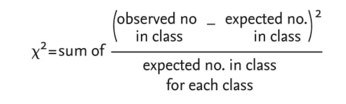# chi-squared test

Also found in: Dictionary, Encyclopedia, Wikipedia.

## chi-squared test

a statistical routine which is a test of SIGNIFICANCE, comparing the observed results of an experiment or sample against the numbers expected from a theory or prediction. The test produces a value called chi-squared (χ2) which is:The χ2 number is then converted to a probability value (P) using an χ2 table. If the P value is larger than 5% we can conclude that there is ‘no significant difference’ between the observed results and those expected, any deviation being due to chance. If, however, the probability is less than 5%, it must be concluded that there is a ‘significant difference’ between the observed results and those expected from theory. Note that the χ2test can only be used with data that fall into discrete categories, e.g. heads or tails, long or short, yellow or orange. Take, for example, a sample of 100 plants arising from a cross between two hybrid red parents. Three quarters of the offspring are expected to be red-flowered, one quarter white. The χ2analysis is shown in the table below.

In this example, there are two classes of data (n = 2) so there is one ‘degree of freedom’ (n - 1). Using the Table of χ2 shows that, with one degree of freedom, a χ2 value of 2.61 indicates a greater than 5% chance that the deviation between observed and expected numbers was due to chance alone, i.e. there is no significant difference between the numbers observed and those expected.

Collins Dictionary of Biology, 3rd ed. © W. G. Hale, V. A. Saunders, J. P. Margham 2005
References in periodicals archive ?
Considering that chi-squared test is used more than the KS test for Benford's Law application in audit scope and in research about frauds, we chose chi-squared.
Factors Affecting Gastrointestinal Haemorrhage, Results of Chi-Squared Test
In Table 2, two different statistical tests were used, a: The Fisher's Exact chi-squared test was used the frequency of observations in each cell were with small sample size (lesser than 5) and b:Yates-corrected chi-squared test was used for the cells which contained sample sizes were larger than 5.
In the preperitoneal group, 54.7% were male and 45.3 were female; the difference was not significant according to Chi-Squared test (p = 0.245) (Table 1).
Changes in resistance trends over time were assessed with chi-squared tests using Stata 10.
The numbers of wet and dry days for 1999-2008 are again predicted and compared to actual data via the chi-squared test, this time finding no significant variation.
For this set of data, you might use a chi-squared test to find out if these four groups are the same.
Pearson was pre-occupied with between-group speciation and thus his statistical methods, such as the Chi-squared test, are categorical in nature.
A chi-squared test for independence found a significant difference between responses to three survey questions asked after each of the three treatments at the 95% confidence level.
We used the Pearson chi-squared test (p<0.05) to compare the bleeding rates in the two groups.
The chi-squared test shows that this degree of variation is probably not the result of chance variations, and we should indeed conclude that Chronicles is significantly sparser in verbs than Kings or Samuel.
We would recommend using tests like the chi-squared test or multivariate analysis to find which factor effects the mosquito population density the most.

Site: Follow: Share:
Open / Close